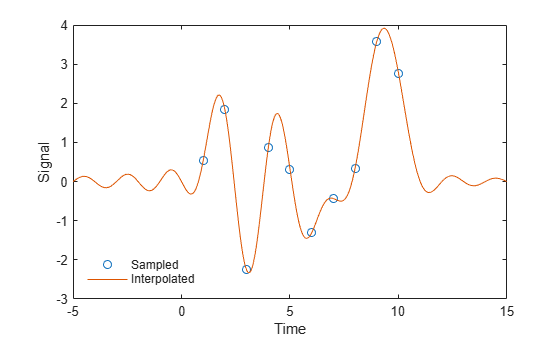sinc

Description

example

y = sinc(x) returns an array, y, whose elements are the sinc of the elements of the input, x. The output y is the same size as x.

Examples

collapse all

Perform ideal bandlimited interpolation of a random signal sampled at integer spacings.

Assume that the signal to interpolate, x, is 0 outside of the given time interval and has been sampled at the Nyquist frequency. Reset the random number generator for reproducibility.

rng default

t = 1:10;
x = randn(size(t))';
ts = linspace(-5,15,600);
[Ts,T] = ndgrid(ts,t);
y = sinc(Ts - T)*x;

plot(t,x,'o',ts,y)
xlabel Time, ylabel Signal
legend('Sampled','Interpolated','Location','SouthWest')
legend boxoffInput Arguments

collapse all

Input array, specified as a real-valued or complex-valued scalar, vector, matrix, N-D array, or gpuArray object. When x is nonscalar, sinc is an element-wise operation.

See Run MATLAB Functions on a GPU (Parallel Computing Toolbox) and GPU Support by Release (Parallel Computing Toolbox) for details on gpuArray (Parallel Computing Toolbox) objects.

Data Types: single | double
Complex Number Support: Yes

Output Arguments

collapse all

Sinc of the input array, x, returned as a real-valued or complex-valued scalar, vector, matrix, N-D array, or gpuArray object of the same size as x.

collapse all

sinc

The sinc function is defined by

$\mathrm{sinc}t=\left\{\begin{array}{cc}\frac{\mathrm{sin}\pi t}{\pi t}& t\ne 0,\\ 1& t=0.\end{array}$

This analytic expression corresponds to the continuous inverse Fourier transform of a rectangular pulse of width 2π and height 1:

$\mathrm{sinc}t=\frac{1}{2\pi }\text{\hspace{0.17em}}\text{\hspace{0.17em}}{\int }_{-\pi }^{\pi }{e}^{j\omega t}\text{\hspace{0.17em}}d\omega .$

The space of functions bandlimited in the frequency range $\omega =\left(-\pi ,\pi \right]$ is spanned by the countably infinite set of sinc functions shifted by integers. Thus, you can reconstruct any such bandlimited function g(t) from its samples at integer spacings:

$g\left(t\right)=\sum _{n=-\infty }^{\infty }g\left(n\right)\mathrm{sinc}\left(t-n\right).$